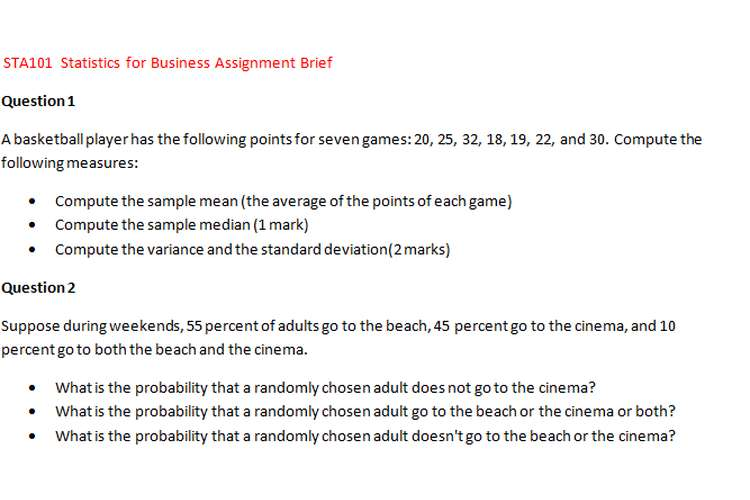This assignment is STA101 statics business assignment brief in which we discuss solution of the statics math topic.

Question 1

A basketball player has the following points for seven games: 20, 25, 32, 18, 19, 22, and 30. Compute the following measures:

• Compute the sample mean (the average of the points of each game)
• Compute the sample median (1 mark)
• Compute the variance and the standard deviation(2 marks)

Question 2

Suppose during weekends, 55 percent of adults go to the beach, 45 percent go to the cinema, and 10 percent go to both the beach and the cinema.

• What is the probability that a randomly chosen adult does not go to the cinema?
• What is the probability that a randomly chosen adult go to the beach or the cinema or both?
• What is the probability that a randomly chosen adult doesn't go to the beach or the cinema?

Question 3:

A Financial Consultant has classified his clients according to their gender and the composition of their investment portfolio (primarily bonds, primarily stocks, or a balanced mix of bonds and stocks). The proportions of clients falling into the various categories are shown in the following table:

 Gender Bonds Stock Balanced Male 0.18 0.20 0.25 Female 0.12 0.16 0.15

One client is selected at random, and two events A and B are defined as follows:

• The client selected is male.
• The client selected has a balanced portfolio. Find the following probabilities:

Find the following probabilities

• P(A)
• P(B)
• P(A or B)
• P(A or B)
• P(A/B)

Question 4

Find the following probabilities by checking the z table

• P(-1.52 < Z < 0.7)
• P((1.15 < Z < 2.45)
• P(-0.9 < Z < -0.3)

Question 5

The foreman of a bottling plant has observed that the amount of soda in each “32-ounce” bottle is actually a normally distributed random variable, with a mean of 32.2 ounces and a standard deviation of 0.3 ounce.

• If a customer buys one bottle, what is the probability that the bottle will contain more than 32 ounces?
• If a customer buys a carton of four bottles, what is the probability that the mean amount of the four bottles will be greater than 32 ounces?